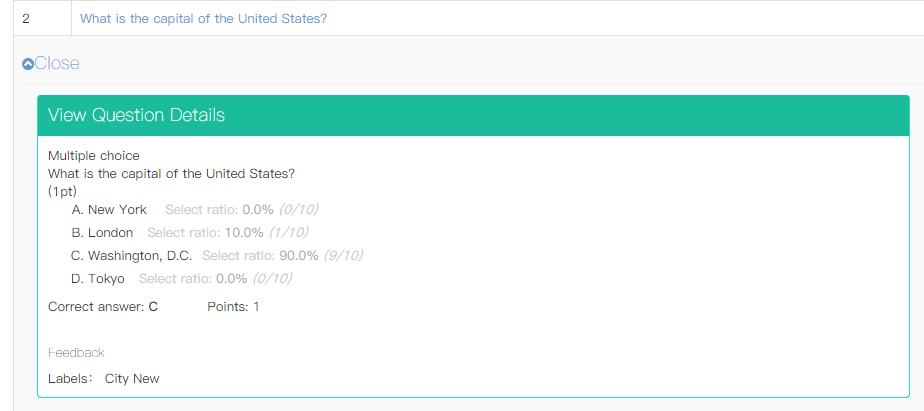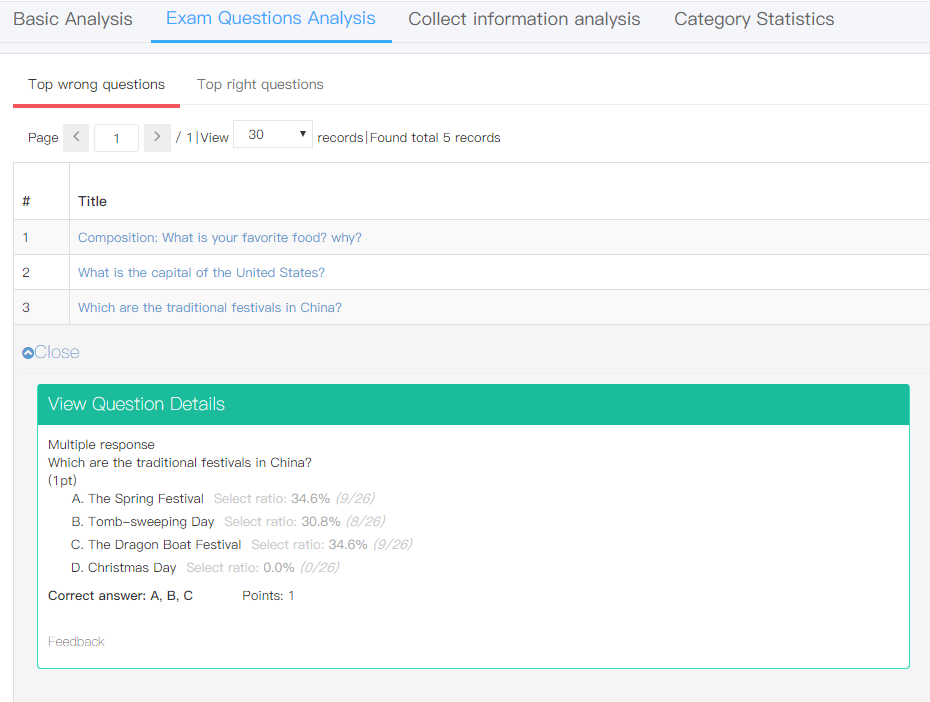# Exam System Calculation on Select Ratio for Choice Questions¶

How does our Online exam system calculate the select ratio for the type of choice questions? The illustration is divided in 2 parts, single choice and multiple choice.

1.Single choice question Formula: Select Ratio = selected times for this option / total taken times for this question For example: Please refer to the question in the picture below:In this question, option B has been selected for 1 time, option C has been selected for 9 times. And this question has been answered for 10 times. The select ratio for option B is 10%. The select ratio for option C is 90%.

2.Multiple choice question Differs from the single choice question, the select ratio is relative to the total selected times for all the options. ***Formula: Select Ratio = selected times for this option / total selected times for all options*** When you enter the exam questions analysis, the select ratio will be shown in the question for your reference:In order to make you more clear about it, please check an example illustration: For example: In a multiple choice question, there are 4 choices. Total 3 candidates have answered this question. Tony has selected options ABC. Tom has selected BCD. Amy has selected BC. So the options they have selected totally are **8 ( =3+3+2). ** Option A has been selected for 1 time. Select ratio for A = ⅛ = 12.5% Option B has been selected for 3 times. Select ratio for B = ⅜ = 37.5% Option C has been selected for 3 times. Select ratio for C = ⅜ = 37.5% Option D has been selected for 1 time. Select ratio for D = ⅛ = 12.5%

Hope this passage is understandable. If you still have any questions, you can sign up and leave us a comment. We are happy to solve problem for you.